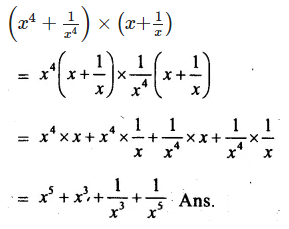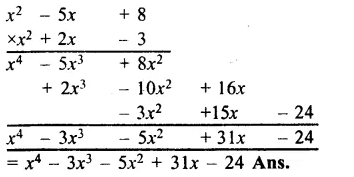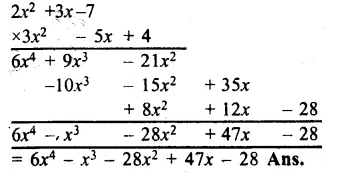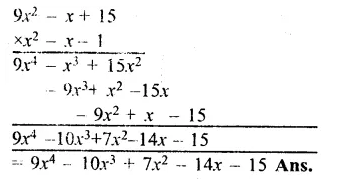## RS Aggarwal Class 8 Solutions Chapter 6 Operations on Algebraic Expressions Ex 6B

These Solutions are part of RS Aggarwal Solutions Class 8. Here we have given RS Aggarwal Solutions Class 8 Chapter 6 Operations on Algebraic Expressions Ex 6B.

Other Exercises

Find each of the following products?

Question 1.
Solution:
(5x + 7) X (3x + 4) = 5x(3x + 4) + 7(3x + 4)
= 15x2 + 20x + 21x + 28 = 15x2 + 41x + 28 Ans.

Question 2.
Solution:
(4x + 9) X (x – 6) = 4x(x – 6) + 9(x – 6)
= 4x2 – 24x + 9x – 54
= 4x2 – 15x – 54 Ans.

Question 3.
Solution:
(2x + 5) X (4x – 3) = 2x(4x – 3) + 5(4x – 3)
= 8x2 – 6x + 20x – 15
= 8x2 + 14x – 15 Ans.

Question 4.
Solution:
(3y – 8) X (5y – 1) = 3y(5y – 1) – 8(5y – 1)
= 15y2 – 3y – 40y + 8
= 15y2 – 43y + 8 Ans.

Question 5.
Solution:
(7x + 2y) X (x + 4y)
= 7x(x + 4y) + 2y(x + 4y)
= 7x2 + 28xy + 2xy + 8y2
= 7x2 + 30xy + 8y2 Ans.

Question 6.
Solution:
(9x + 5y) X (4x + 3y) = 9x(4x + 3y) + 5y(4x + 3y)
= 36x2 + 27xy + 20xy + 15y2
= 36x2 + 47xy + 15y2 Ans.

Question 7.
Solution:
(3m – 4n) X (2m – 3n)
= 3m(2m – 3n) – 4n(2m – 3n)
= 6m2 – 9mn – 8mn + 12n2
= 6m2 – 17mn + 12n2 Ans.

Question 8.
Solution:
(x2 – a2) X (x – a)
= x2(x – a) – a2(x – a)
= x3 – x2a – xa2 + a3 Ans.

Question 9.
Solution:
(x2 – y2) X (x + 2y)
= x2(x + 2y) – y2(x + 2y)
= x3 + 2x2y – xy2 – 2y3 Ans.

Question 10.
Solution:
(3p2 + q2) X (2p2 – 3q2)
= 3p2(2p2 – 3q2) + q2(2p2 – 3q2)
= 6p4 – 9p2q2 + 2p2q2 – 3q4
= 6p4 – 7p2q2 – 3q4 Ans.

Question 11.
Solution:
(2x2 – 5y2) X (x2 + 3y2)
= 2x2(x2 + 3y2) – 5y2(x2 + 3y2)
= 2x4 + 6x2y2 – 5x2y2 – 15y4)
= 2x4 + x2y2 – 15y4 Ans.

Question 12.
Solution:
(x3 – y3) X (x2 + y2)
= x3(x2 + y2) – y3(x2 + y2)
= x5 + x3y2 – x2y3 – y5 Ans.

Question 13.
Solution:
(x4 + y4) X (x2 – y2)
= x4(x2 – y2) + y4(x2 – y2)
= x6 – x4y2 + x2y4 – y6 Ans.

Question 14.
Solution:
$$\left( { x }^{ 4 }+\frac { 1 }{ { x }^{ 4 } } \right) \times \left( { x+ }\frac { 1 }{ { x } } \right)$$Find each of the following products:

Question 15.
Solution:
(x2 – 3x + 7) X (2x + 3)
= (x2 – 3x + 7) (2x) + (x2 – 3x + 7) X 3
= 2x3 – 6x + 14x + 3x2 – 9x + 21
= 2x3 – 3x2 + 5x + 21 Ans.

Question 16.
Solution:
(3x2 + 5x – 9) X (3x – 5)
= 3x2(3x – 5x) + 5x(3x – 5) – 9(3x – 5)
= 9x3 – 15x2 + 15x2 – 25x – 27x + 45
= 9x3 – 52x + 45 Ans.

Question 17.
Solution:
(x2 – xy + y2) X (x + y)
= (x2 – xy + y2) X x + (x2 – xy + y2) X y
= x3 – x2y + xy2 + x2y – xy2 + y3
= x3 + y3 Ans.

Question 18.
Solution:
(x2 + xy + y2) X (x – y)
(x2 + xy + y2) X x + (x2 + xy + y2) X ( – y)
= x3 + x2y + xy2 – x2y – xy2 – y3
= x3 – y3 Ans.

Question 19.
Solution:
(x3 – 2x2 + 5) X (4x – 1)
= (x3 – 2x2 + 5) X 4x + (x3 – 2x2 + 5) X ( – 1)
= 4x4 – 8x3 + 20x – x3 + 2x2 – 5
= 4x4 – 9x3 + 2x2 + 20x – 5 Ans.

Question 20.
Solution:
(9x2 – x + 15) X (x2 – 3)
(9x2 – X + 15) X x2 + (9x2 – x + 15) X ( – 3)
= 9x4 – x3 + 15x2 – 27x2 + 3x – 45
= 9x4 – x3 – 12x2 + 3x – 45 Ans.

Question 21.
Solution:
(x2 – 5x + 8) X (x2 + 2)
= (x2 – 5x + 8) X x2 + (x2 – 5x + 8) X 2
= x4 – 5x3 + 8x2 + 2x2 – 10x + 16
= x4 – 5x3 + 10x2 – 10x + 16 Ans.

Question 22.
Solution:
(x3 – 5x2 + 3x + 1) X (x2 – 3)
= (x3 – 5x2 + 3x + 1) X x2 + (x3 – 5x2 + 3x + 1) X ( – 3)
= x5 – 5x4 + 3x3 + x2 – 3x3 + 15x2 – 9x – 3
= x5 – 5x4 + 16x2 – 9x – 3 Ans.

Question 23.
Solution:
(3x + 2y – 4) X (x – y + 2)Question 24.
Solution:
(x2 – 5x + 8) X (x2 + 2x – 3)Question 25.
Solution:
(2x2 + 3x – 7) X (3x2 – 5x + 4)Question 26.
Solution:
(9x2 – x + 15) X (x2 – x – 1)Hope given RS Aggarwal Solutions Class 8 Chapter 6 Operations on Algebraic Expressions Ex 6B are helpful to complete your math homework.

If you have any doubts, please comment below. Learn Insta try to provide online math tutoring for you.